# Ionic equilibrium part 1

Teacher
28 de May de 2021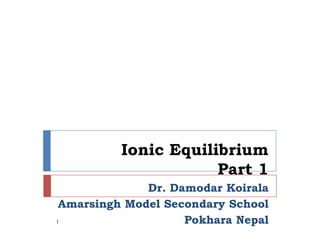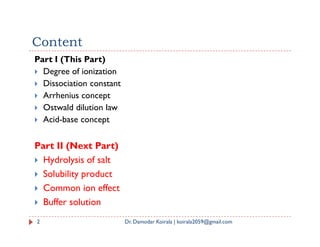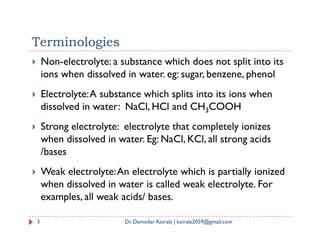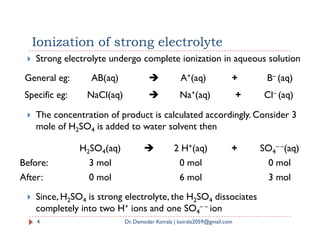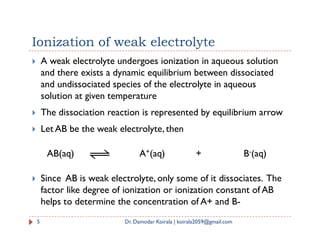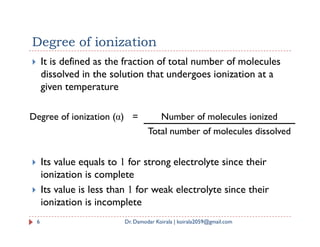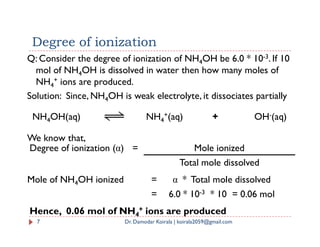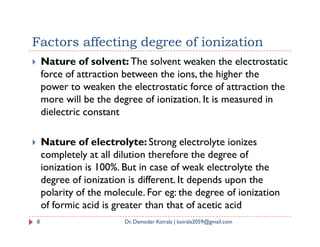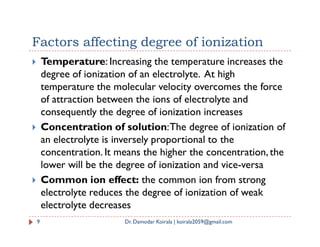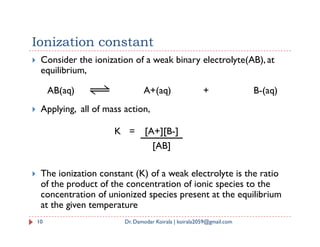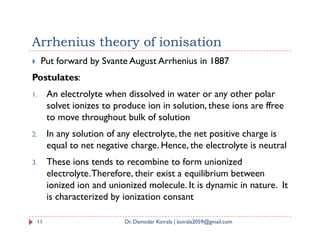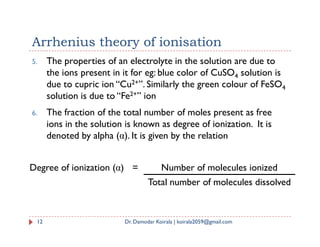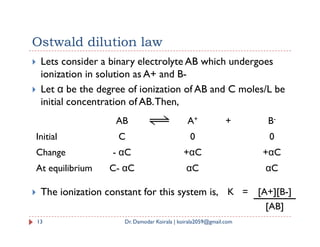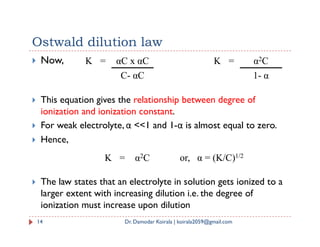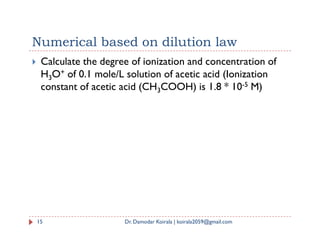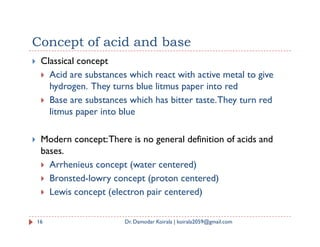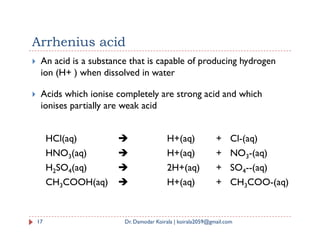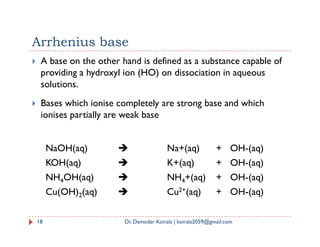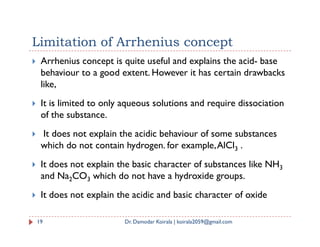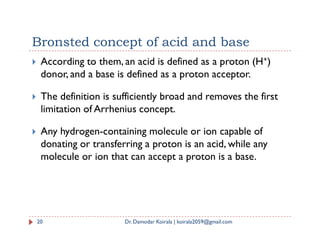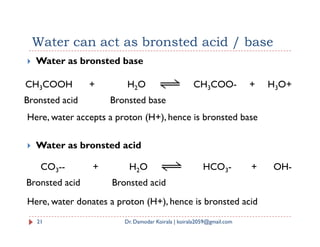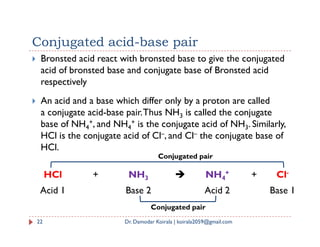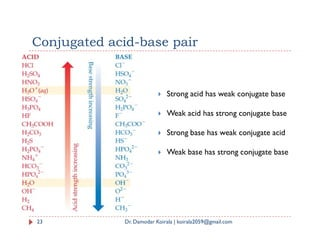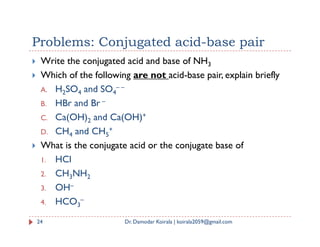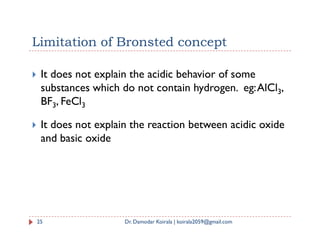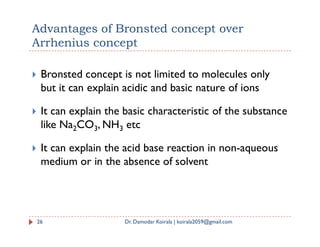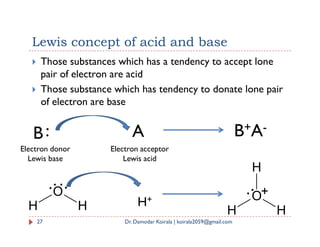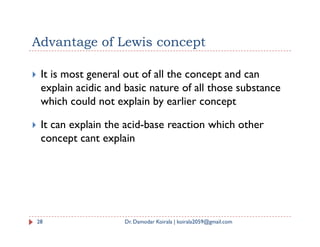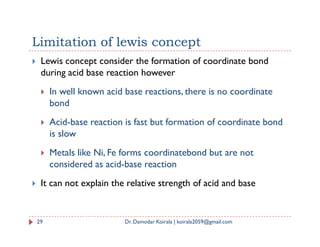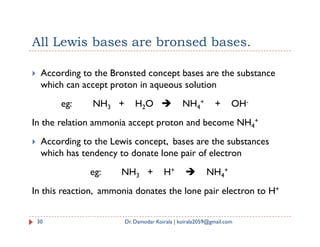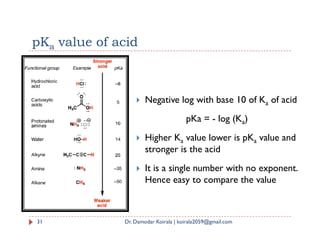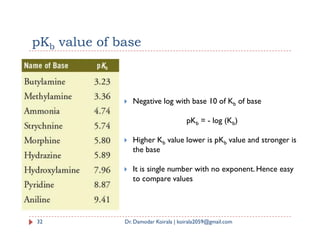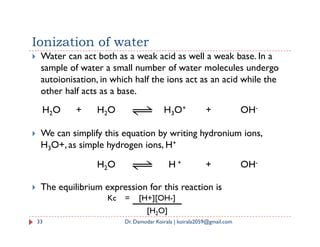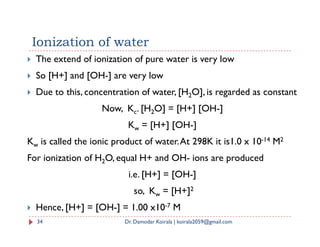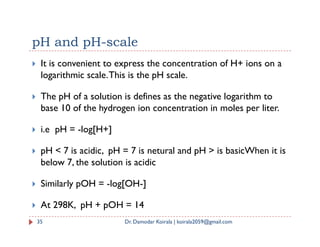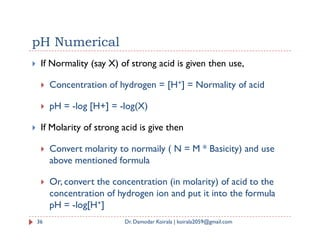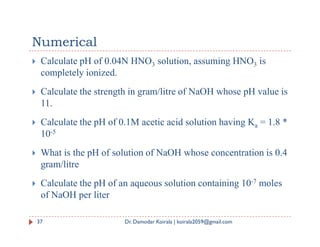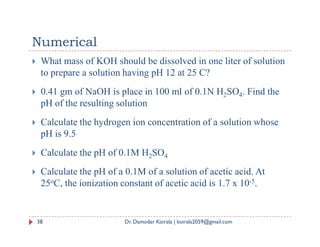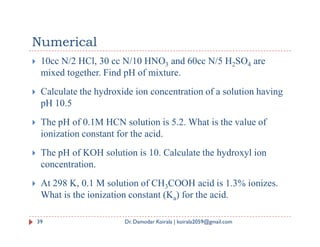1 de 39

### Ionic equilibrium part 1

• 1. Ionic Equilibrium Part 1 Dr. Damodar Koirala Amarsingh Model Secondary School Pokhara Nepal 1
• 2. Content Part I (This Part)  Degree of ionization  Dissociation constant  Arrhenius concept  Ostwald dilution law  Acid-base concept 2 Dr. Damodar Koirala | koirala2059@gmail.com  Acid-base concept Part II (Next Part)  Hydrolysis of salt  Solubility product  Common ion effect  Buffer solution
• 3. Terminologies  Non-electrolyte: a substance which does not split into its ions when dissolved in water. eg: sugar, benzene, phenol  Electrolyte:A substance which splits into its ions when dissolved in water: NaCl, HCl and CH3COOH  Strong electrolyte: electrolyte that completely ionizes  Strong electrolyte: electrolyte that completely ionizes when dissolved in water. Eg: NaCl, KCl, all strong acids /bases  Weak electrolyte:An electrolyte which is partially ionized when dissolved in water is called weak electrolyte. For examples, all weak acids/ bases. 3 Dr. Damodar Koirala | koirala2059@gmail.com
• 4. Ionization of strong electrolyte  Strong electrolyte undergo complete ionization in aqueous solution  The concentration of product is calculated accordingly. Consider 3 mole of H SO is added to water solvent then General eg: AB(aq)  A+(aq) + B– (aq) Specific eg: NaCl(aq)  Na+(aq) + Cl– (aq) mole of H2SO4 is added to water solvent then  Since, H2SO4 is strong electrolyte, the H2SO4 dissociates completely into two H+ ions and one SO4 – – ion 4 Dr. Damodar Koirala | koirala2059@gmail.com H2SO4(aq)  2 H+(aq) + SO4 – –(aq) Before: 3 mol 0 mol 0 mol After: 0 mol 6 mol 3 mol
• 5. Ionization of weak electrolyte  A weak electrolyte undergoes ionization in aqueous solution and there exists a dynamic equilibrium between dissociated and undissociated species of the electrolyte in aqueous solution at given temperature  The dissociation reaction is represented by equilibrium arrow Let AB be the weak electrolyte, then  Let AB be the weak electrolyte, then  Since AB is weak electrolyte, only some of it dissociates. The factor like degree of ionization or ionization constant of AB helps to determine the concentration of A+ and B- 5 Dr. Damodar Koirala | koirala2059@gmail.com AB(aq) A+(aq) + B-(aq)
• 6.  It is defined as the fraction of total number of molecules dissolved in the solution that undergoes ionization at a given temperature Degree of ionization Degree of ionization (α) = Number of molecules ionized  Its value equals to 1 for strong electrolyte since their ionization is complete  Its value is less than 1 for weak electrolyte since their ionization is incomplete Total number of molecules dissolved 6 Dr. Damodar Koirala | koirala2059@gmail.com
• 7. NH4OH(aq) NH4 +(aq) + OH-(aq) Q: Consider the degree of ionization of NH4OH be 6.0 * 10-3. If 10 mol of NH4OH is dissolved in water then how many moles of NH4 + ions are produced. Solution: Since, NH4OH is weak electrolyte, it dissociates partially Degree of ionization We know that, Degree of ionization (α) = Mole ionized Total mole dissolved 7 Dr. Damodar Koirala | koirala2059@gmail.com Mole of NH4OH ionized = α * Total mole dissolved = 6.0 * 10-3 * 10 = 0.06 mol Hence, 0.06 mol of NH4 + ions are produced
• 8. Factors affecting degree of ionization  Nature of solvent: The solvent weaken the electrostatic force of attraction between the ions, the higher the power to weaken the electrostatic force of attraction the more will be the degree of ionization. It is measured in dielectric constant  Nature of electrolyte: Strong electrolyte ionizes completely at all dilution therefore the degree of ionization is 100%. But in case of weak electrolyte the degree of ionization is different. It depends upon the polarity of the molecule. For eg: the degree of ionization of formic acid is greater than that of acetic acid 8 Dr. Damodar Koirala | koirala2059@gmail.com
• 9. Factors affecting degree of ionization  Temperature: Increasing the temperature increases the degree of ionization of an electrolyte. At high temperature the molecular velocity overcomes the force of attraction between the ions of electrolyte and consequently the degree of ionization increases  Concentration of solution:The degree of ionization of  Concentration of solution:The degree of ionization of an electrolyte is inversely proportional to the concentration. It means the higher the concentration, the lower will be the degree of ionization and vice-versa  Common ion effect: the common ion from strong electrolyte reduces the degree of ionization of weak electrolyte decreases 9 Dr. Damodar Koirala | koirala2059@gmail.com
• 10. Ionization constant  Consider the ionization of a weak binary electrolyte(AB), at equilibrium,  Applying, all of mass action, AB(aq) A+(aq) + B-(aq)  The ionization constant (K) of a weak electrolyte is the ratio of the product of the concentration of ionic species to the concentration of unionized species present at the equilibrium at the given temperature 10 Dr. Damodar Koirala | koirala2059@gmail.com K = [A+][B-] [AB]
• 11. Arrhenius theory of ionisation  Put forward by Svante August Arrhenius in 1887 Postulates: 1. An electrolyte when dissolved in water or any other polar solvet ionizes to produce ion in solution, these ions are ffree to move throughout bulk of solution 2. In any solution of any electrolyte, the net positive charge is equal to net negative charge. Hence, the electrolyte is neutral 3. These ions tends to recombine to form unionized electrolyte.Therefore, their exist a equilibrium between ionized ion and unionized molecule. It is dynamic in nature. It is characterized by ionization consant 11 Dr. Damodar Koirala | koirala2059@gmail.com
• 12. Arrhenius theory of ionisation 5. The properties of an electrolyte in the solution are due to the ions present in it for eg: blue color of CuSO4 solution is due to cupric ion “Cu2+”. Similarly the green colour of FeSO4 solution is due to “Fe2+” ion 6. The fraction of the total number of moles present as free ions in the solution is known as degree of ionization. It is ions in the solution is known as degree of ionization. It is denoted by alpha (α). It is given by the relation 12 Dr. Damodar Koirala | koirala2059@gmail.com Degree of ionization (α) = Number of molecules ionized Total number of molecules dissolved
• 13. Ostwald dilution law  Lets consider a binary electrolyte AB which undergoes ionization in solution as A+ and B-  Let α be the degree of ionization of AB and C moles/L be initial concentration of AB.Then, AB A+ + B-  The ionization constant for this system is, 13 Dr. Damodar Koirala | koirala2059@gmail.com AB A + B Initial C 0 0 Change - αC +αC +αC At equilibrium C- αC αC αC K = [A+][B-] [AB]
• 14. Ostwald dilution law  Now, K = αC x αC C- αC K = α2C 1- α  This equation gives the relationship between degree of ionization and ionization constant.  For weak electrolyte, α <<1 and 1-α is almost equal to zero. 14 Dr. Damodar Koirala | koirala2059@gmail.com  For weak electrolyte, α <<1 and 1-α is almost equal to zero.  Hence,  The law states that an electrolyte in solution gets ionized to a larger extent with increasing dilution i.e. the degree of ionization must increase upon dilution K = α2C or, α = (K/C)1/2
• 15. Numerical based on dilution law  Calculate the degree of ionization and concentration of H3O+ of 0.1 mole/L solution of acetic acid (Ionization constant of acetic acid (CH3COOH) is 1.8 * 10-5 M) 15 Dr. Damodar Koirala | koirala2059@gmail.com
• 16. Concept of acid and base  Classical concept  Acid are substances which react with active metal to give hydrogen. They turns blue litmus paper into red  Base are substances which has bitter taste.They turn red litmus paper into blue  Modern concept:There is no general definition of acids and bases.  Arrhenieus concept (water centered)  Bronsted-lowry concept (proton centered)  Lewis concept (electron pair centered) 16 Dr. Damodar Koirala | koirala2059@gmail.com
• 17. Arrhenius acid  An acid is a substance that is capable of producing hydrogen ion (H+ ) when dissolved in water  Acids which ionise completely are strong acid and which ionises partially are weak acid 17 Dr. Damodar Koirala | koirala2059@gmail.com HCl(aq)  H+(aq) + Cl-(aq) HNO3(aq)  H+(aq) + NO3-(aq) H2SO4(aq)  2H+(aq) + SO4--(aq) CH3COOH(aq)  H+(aq) + CH3COO-(aq)
• 18. Arrhenius base  A base on the other hand is defined as a substance capable of providing a hydroxyl ion (HO) on dissociation in aqueous solutions.  Bases which ionise completely are strong base and which ionises partially are weak base 18 Dr. Damodar Koirala | koirala2059@gmail.com NaOH(aq)  Na+(aq) + OH-(aq) KOH(aq)  K+(aq) + OH-(aq) NH4OH(aq)  NH4+(aq) + OH-(aq) Cu(OH)2(aq)  Cu2+(aq) + OH-(aq)
• 19. Limitation of Arrhenius concept  Arrhenius concept is quite useful and explains the acid- base behaviour to a good extent. However it has certain drawbacks like,  It is limited to only aqueous solutions and require dissociation of the substance.  It does not explain the acidic behaviour of some substances which do not contain hydrogen. for example,AlCl3 .  It does not explain the basic character of substances like NH3 and Na2CO3 which do not have a hydroxide groups.  It does not explain the acidic and basic character of oxide 19 Dr. Damodar Koirala | koirala2059@gmail.com
• 20. Bronsted concept of acid and base  According to them, an acid is defined as a proton (H+) donor, and a base is defined as a proton acceptor.  The definition is sufficiently broad and removes the first limitation of Arrhenius concept.  Any hydrogen-containing molecule or ion capable of donating or transferring a proton is an acid, while any molecule or ion that can accept a proton is a base. 20 Dr. Damodar Koirala | koirala2059@gmail.com
• 21. Water can act as bronsted acid / base  Water as bronsted base Here, water accepts a proton (H+), hence is bronsted base CH3COOH + H2O CH3COO- + H3O+ Bronsted acid Bronsted base  Water as bronsted acid Here, water donates a proton (H+), hence is bronsted acid 21 Dr. Damodar Koirala | koirala2059@gmail.com CO3-- + H2O HCO3- + OH- Bronsted acid Bronsted acid
• 22. Conjugated acid-base pair  Bronsted acid react with bronsted base to give the conjugated acid of bronsted base and conjugate base of Bronsted acid respectively  An acid and a base which differ only by a proton are called a conjugate acid-base pair.Thus NH3 is called the conjugate base of NH4 +, and NH4 + is the conjugate acid of NH3. Similarly, base of NH4 +, and NH4 + is the conjugate acid of NH3. Similarly, HCl is the conjugate acid of Cl–, and Cl– the conjugate base of HCl. 22 Dr. Damodar Koirala | koirala2059@gmail.com HCl + NH3  NH4 + + Cl- Acid 1 Base 2 Acid 2 Base 1 Conjugated pair Conjugated pair
• 23. Conjugated acid-base pair  Strong acid has weak conjugate base  Weak acid has strong conjugate base 23 Dr. Damodar Koirala | koirala2059@gmail.com  Strong base has weak conjugate acid  Weak base has strong conjugate base
• 24. Problems: Conjugated acid-base pair  Write the conjugated acid and base of NH3  Which of the following are not acid-base pair, explain briefly A. H2SO4 and SO4 – – B. HBr and Br – C. Ca(OH)2 and Ca(OH)+ 2 D. CH4 and CH5 +  What is the conjugate acid or the conjugate base of 1. HCl 2. CH3NH2 3. OH– 4. HCO3 – 24 Dr. Damodar Koirala | koirala2059@gmail.com
• 25. Limitation of Bronsted concept  It does not explain the acidic behavior of some substances which do not contain hydrogen. eg:AlCl3, BF3, FeCl3  It does not explain the reaction between acidic oxide  It does not explain the reaction between acidic oxide and basic oxide 25 Dr. Damodar Koirala | koirala2059@gmail.com
• 26. Advantages of Bronsted concept over Arrhenius concept  Bronsted concept is not limited to molecules only but it can explain acidic and basic nature of ions  It can explain the basic characteristic of the substance like Na2CO3, NH3 etc like Na2CO3, NH3 etc  It can explain the acid base reaction in non-aqueous medium or in the absence of solvent 26 Dr. Damodar Koirala | koirala2059@gmail.com
• 27. Lewis concept of acid and base  Those substances which has a tendency to accept lone pair of electron are acid  Those substance which has tendency to donate lone pair of electron are base B: A B+A- 27 Dr. Damodar Koirala | koirala2059@gmail.com B: A B+A- Electron donor Lewis base Electron acceptor Lewis acid H+ O H H O H H H +
• 28. Advantage of Lewis concept  It is most general out of all the concept and can explain acidic and basic nature of all those substance which could not explain by earlier concept  It can explain the acid-base reaction which other  It can explain the acid-base reaction which other concept cant explain 28 Dr. Damodar Koirala | koirala2059@gmail.com
• 29. Limitation of lewis concept  Lewis concept consider the formation of coordinate bond during acid base reaction however  In well known acid base reactions, there is no coordinate bond  Acid-base reaction is fast but formation of coordinate bond  Acid-base reaction is fast but formation of coordinate bond is slow  Metals like Ni, Fe forms coordinatebond but are not considered as acid-base reaction  It can not explain the relative strength of acid and base 29 Dr. Damodar Koirala | koirala2059@gmail.com
• 30. All Lewis bases are bronsed bases.  According to the Bronsted concept bases are the substance which can accept proton in aqueous solution eg: NH3 + H2O  NH4 + + OH- In the relation ammonia accept proton and become NH4 + In the relation ammonia accept proton and become NH4 +  According to the Lewis concept, bases are the substances which has tendency to donate lone pair of electron eg: NH3 + H+  NH4 + In this reaction, ammonia donates the lone pair electron to H+ 30 Dr. Damodar Koirala | koirala2059@gmail.com
• 31. pKa value of acid  Negative log with base 10 of Ka of acid pKa = - log (Ka) pKa = - log (Ka)  Higher Ka value lower is pKa value and stronger is the acid  It is a single number with no exponent. Hence easy to compare the value 31 Dr. Damodar Koirala | koirala2059@gmail.com
• 32. pKb value of base  Negative log with base 10 of Kb of base pKb = - log (Kb) 32 Dr. Damodar Koirala | koirala2059@gmail.com pKb = - log (Kb)  Higher Kb value lower is pKb value and stronger is the base  It is single number with no exponent. Hence easy to compare values
• 33. Ionization of water  Water can act both as a weak acid as well a weak base. In a sample of water a small number of water molecules undergo autoionisation, in which half the ions act as an acid while the other half acts as a base. H2O + H2O H3O+ + OH-  We can simplify this equation by writing hydronium ions, H3O+, as simple hydrogen ions, H+  The equilibrium expression for this reaction is 33 Dr. Damodar Koirala | koirala2059@gmail.com H2O H + + OH- Kc = [H+][OH-] [H2O]
• 34. Ionization of water  The extend of ionization of pure water is very low  So [H+] and [OH-] are very low  Due to this, concentration of water, [H2O], is regarded as constant Now, Kc. [H2O] = [H+] [OH-] K = [H+] [OH-] Kw = [H+] [OH-] Kw is called the ionic product of water.At 298K it is1.0 x 10-14 M2 For ionization of H2O, equal H+ and OH- ions are produced i.e. [H+] = [OH-] so, Kw = [H+]2  Hence, [H+] = [OH-] = 1.00 x10-7 M 34 Dr. Damodar Koirala | koirala2059@gmail.com
• 35. pH and pH-scale  It is convenient to express the concentration of H+ ions on a logarithmic scale.This is the pH scale.  The pH of a solution is defines as the negative logarithm to base 10 of the hydrogen ion concentration in moles per liter. i.e pH = -log[H+]  i.e pH = -log[H+]  pH < 7 is acidic, pH = 7 is netural and pH > is basicWhen it is below 7, the solution is acidic  Similarly pOH = -log[OH-]  At 298K, pH + pOH = 14 35 Dr. Damodar Koirala | koirala2059@gmail.com
• 36. pH Numerical  If Normality (say X) of strong acid is given then use,  Concentration of hydrogen = [H+] = Normality of acid  pH = -log [H+] = -log(X) If Molarity of strong acid is give then  If Molarity of strong acid is give then  Convert molarity to normaily ( N = M * Basicity) and use above mentioned formula  Or, convert the concentration (in molarity) of acid to the concentration of hydrogen ion and put it into the formula pH = -log[H+] 36 Dr. Damodar Koirala | koirala2059@gmail.com
• 37. Numerical  Calculate pH of 0.04N HNO3 solution, assuming HNO3 is completely ionized.  Calculate the strength in gram/litre of NaOH whose pH value is 11.  Calculate the pH of 0.1M acetic acid solution having Ka = 1.8 *  Calculate the pH of 0.1M acetic acid solution having Ka = 1.8 * 10-5  What is the pH of solution of NaOH whose concentration is 0.4 gram/litre  Calculate the pH of an aqueous solution containing 10-7 moles of NaOH per liter 37 Dr. Damodar Koirala | koirala2059@gmail.com
• 38. Numerical  What mass of KOH should be dissolved in one liter of solution to prepare a solution having pH 12 at 25 C?  0.41 gm of NaOH is place in 100 ml of 0.1N H2SO4. Find the pH of the resulting solution  Calculate the hydrogen ion concentration of a solution whose  Calculate the hydrogen ion concentration of a solution whose pH is 9.5  Calculate the pH of 0.1M H2SO4  Calculate the pH of a 0.1M of a solution of acetic acid. At 25oC, the ionization constant of acetic acid is 1.7 x 10-5. 38 Dr. Damodar Koirala | koirala2059@gmail.com
• 39. Numerical  10cc N/2 HCl, 30 cc N/10 HNO3 and 60cc N/5 H2SO4 are mixed together. Find pH of mixture.  Calculate the hydroxide ion concentration of a solution having pH 10.5  The pH of 0.1M HCN solution is 5.2. What is the value of  The pH of 0.1M HCN solution is 5.2. What is the value of ionization constant for the acid.  The pH of KOH solution is 10. Calculate the hydroxyl ion concentration.  At 298 K, 0.1 M solution of CH3COOH acid is 1.3% ionizes. What is the ionization constant (Ka) for the acid. 39 Dr. Damodar Koirala | koirala2059@gmail.com